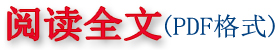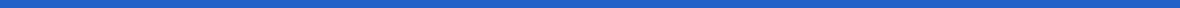阎坤. 地球空间稳定核素的趋势分析方程与物质的超光速运动规律[R]. 西安现代非线性科学应用研究所, 2006 04 26.
 本文框架内容发表在： 阎坤. 地球空间稳定核素的趋势分析方程与物质的超光速运动规律[J]. 地球物理学进展，2006，21(1)：38～47. YAN Kun. The tendency analytical equations of stable nuclides and the superluminal velocity motion laws of matter in geospace[J]. Progress in Geophysics（in Chinese with abstract in English），2006，21(1)：38～47.

# 地球空间稳定核素的趋势分析方程与物质的超光速运动规律

(西安现代非线性科学应用研究所  西安 710061)

 摘  要：通过讨论地球空间已有稳定核素内质子数与中子数的分布趋势，介绍了稳定核素的趋势分析方法及其有关周期性分布方程形式，给出了理论方程曲线与地球空间稳定核素实验数据分布点的对比结果，进而给出了稳定核素极限值和元素周期表中化学元素极限，以及其与正负粒子对的可能对应关系方程，包括位于电子中微子层面附近的粒子质量量级初步估计及粒子质量的趋势性分布方程（具有稠密分布在部分闭区间与稀疏分布在少数开区间的特征）。随后通过建立真空物质能量状态的二个假设，及基于等效Binet方程，给出了与Einstein狭义相对论有关结论相融合的物质粒子以光速及超光速运动的质量及能量方程；作为推论，对这些方程与暗物质及暗能量的可能对应关系予以了初步探讨。 关键词：稳定核素，趋势分析方程，周期性规律，化学元素极限，粒子质量分布，真空物质能量状态，超光速运动方程
 YAN  Kun (Xi’an Modern Nonlinear Science Applying Institute，Xi’an  710061，China)
 Abstract  In this paper, by discussing the existent distribution trend of relation for the proton number and the neutron number to be included by the stable nuclides in geospace, tendency analytical method and it’s periodic distribution equation forms of the stable nuclides are expressed at first. Then a comparison result between the curve of the theoretical equation analysis and the points of the experimental distribution data of the stable nuclides in geospace are given. Further more, stable nuclide limit and chemical element limit for the chemical element periodic table are given, and possible corresponding relation equation with the positron-particle annihilation is expressed, which includes estimation of order of the static mass to be situated nearby at the electron neutrino structural dimension and a tendency distribution equation (with the characteristics of dense distribution in the partial closed intervals and sparse distribution in the few open intervals) of the particles mass. Subsequently, by forming two hypotheses about energy state of vacuum matter, and basing on an equivalent Binet’s equation, mass equations and energy equations of the partial moving with light-velocity or superluminal (also faster-than-light or FTL) velocity motion fusing with the results of Einstein special relativity are expressed. As inference, possible corresponding relations between the mass equations and energy equations with the dark matter and dark energy are discussed tentatively. Keywords stable nuclide, tendency analytical equation, periodic law, chemical element limit, tendency distribution of the particles mass, energy state of vacuum matter, equations of superluminal velocity motion
 引  言 地球空间的化学元素由于Mendeleev、Moseley等人的工作成果已建立出能够说明元素之间渐进关系的周期表，随后人们根据化学元素以及稳定核素的有关性质进行了深入的分析，包括核素之间的合成转化规律等研究，给出了β稳定核素核子数A与其中质子数Z 的一典型关系方程...相关研究